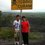# Momentum and its relation to Force

Sir Isaac Newton First proposed that $\vec{F} = \frac{d\vec{p}}{dt}$ before he proposed that $\vec{F} = m\vec{a}$ from the first formula it is easy to prove the second.

$\vec{F} = \frac{d\vec{p}}{dt}$

$\vec{F} = \frac{d(m\vec{v})}{dt}$

$\vec{F} = m\frac{d\vec{v}}{dt}+\vec{v}\frac{dm}{dt}$

From the definition of acceleration and assuming mass to be a constant,

$\vec{F} = m\vec{a}$

However first of all the books always prove that $\vec{F} = m\vec{a}$ as because acceleration is directly proportional to force and inversely proportional to mass. From $\vec{F}=m\vec{a}$ they then prove that $\vec{F} = \frac{d\vec{p}}{dt}$

I have a few problems with this. The book starts by saying the following.

$\vec{F} = m\vec{a}$

$\vec{F} = m\frac{d\vec{v}}{dt}$

They say since mass is a constant it can be moved into the derivative. (Remember the fact that mass is a constant)

$\vec{F} = \frac{d(m\vec{v})}{dt}$

$\vec{F} = \frac{d\vec{p}}{dt}$

From here they conclude that hence we can use the product rule and find force for varying mass (e.g. rockets using up fuel)

$\vec{F} = \frac{d\vec{p}}{dt} = \frac{d(m\vec{v})}{dt} = m\frac{d\vec{v}}{dt} + \vec{v}\frac{dm}{dt}$

this doesn't make sense as in the proof they said mass is constant and that is why u can have $m\frac{d\vec{v}}{dt} = \frac{d(m\vec{v})}{dt}$. If mass was varying we can't do that. So can someone give me the right proof.

Secondly and lastly, how did Newton show $\vec{F} = \frac{d\vec{p}}{dt}$ before he showed Newton's Second Law. Whats the proof?6 years, 8 months ago

This discussion board is a place to discuss our Daily Challenges and the math and science related to those challenges. Explanations are more than just a solution — they should explain the steps and thinking strategies that you used to obtain the solution. Comments should further the discussion of math and science.

When posting on Brilliant:

• Use the emojis to react to an explanation, whether you're congratulating a job well done , or just really confused .
• Ask specific questions about the challenge or the steps in somebody's explanation. Well-posed questions can add a lot to the discussion, but posting "I don't understand!" doesn't help anyone.
• Try to contribute something new to the discussion, whether it is an extension, generalization or other idea related to the challenge.

MarkdownAppears as
*italics* or _italics_ italics
**bold** or __bold__ bold
- bulleted- list
• bulleted
• list
1. numbered2. list
1. numbered
2. list
Note: you must add a full line of space before and after lists for them to show up correctly
paragraph 1paragraph 2

paragraph 1

paragraph 2

[example link](https://brilliant.org)example link
> This is a quote
This is a quote
    # I indented these lines
# 4 spaces, and now they show
# up as a code block.

print "hello world"
# I indented these lines
# 4 spaces, and now they show
# up as a code block.

print "hello world"
MathAppears as
Remember to wrap math in $$ ... $$ or $ ... $ to ensure proper formatting.
2 \times 3 $2 \times 3$
2^{34} $2^{34}$
a_{i-1} $a_{i-1}$
\frac{2}{3} $\frac{2}{3}$
\sqrt{2} $\sqrt{2}$
\sum_{i=1}^3 $\sum_{i=1}^3$
\sin \theta $\sin \theta$
\boxed{123} $\boxed{123}$